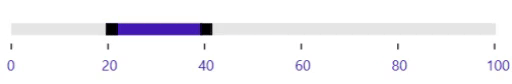# Range in WPF Range Slider (SfRangeSlider)

7 May 20219 minutes to read

The SfRangeSlider control provides support to select the range of value using two thumbs.

## ShowRange

When ShowRange property is set to true, two thumbs are placed in the track. One thumb is used to update the start of the range selection and another thumb is used to update the end of the range selection.

``````<editors:SfRangeSlider
Width="300"
Maximum="100"
Minimum="0"
RangeEnd="70"
RangeStart="40"
ShowRange="True" />``````
``````Grid parentGrid = new Grid();
SfRangeSlider rangeSlider = new SfRangeSlider()
{
Width = 300,
Maximum = 100,
Minimum = 0,
ShowRange = true,
RangeStart = 40,
RangeEnd = 70
};

this.Content = parentGrid;``````NOTE

When the `ShowRange` property is set to true, both ToolTip will display RangeStart and RangeEnd.

## RangeStart

Gets or sets the start value of the range start.

``````<editors:SfRangeSlider
Width="300"
Maximum="100"
Minimum="0"
RangeEnd="70"
RangeStart="40"
ShowRange="True" />``````
``````Grid parentGrid = new Grid();
SfRangeSlider rangeSlider = new SfRangeSlider()
{
Width = 300,
Maximum = 100,
Minimum = 0,
ShowRange = true,
RangeStart = 40,
RangeEnd = 70
};

this.Content = parentGrid;``````## RangeEnd

Gets or sets the end value of the range end.

``````<editors:SfRangeSlider
Width="300"
Maximum="100"
Minimum="0"
RangeEnd="70"
RangeStart="40"
ShowRange="True" />``````
``````Grid parentGrid = new Grid();
SfRangeSlider rangeSlider = new SfRangeSlider()
{
Width = 300,
Maximum = 100,
Minimum = 0,
ShowRange = true,
RangeStart = 40,
RangeEnd = 70
};

this.Content = parentGrid;``````## Drag Selected Range

The `AllowRangeDrag` API allows the range in the Range Slider to be adjusted and the range to be dragged without changing the start and end ranges individually. The default value of `AllowRangeDrag` is false.

``````<editors:SfRangeSlider
Width="300"
AllowRangeDrag="True"
Maximum="100"
Minimum="0"
RangeEnd="20"
RangeStart="0"
ShowRange="True"
ShowValueLabels="True"
TickFrequency="20" />``````
``````Grid parentGrid = new Grid();
SfRangeSlider rangeSlider = new SfRangeSlider()
{
Width = 300,
Maximum = 100,
Minimum = 0,
ShowRange = true,
RangeStart = 0,
RangeEnd = 20,
ShowValueLabels = true,
TickFrequency = 20,
AllowRangeDrag = true
};